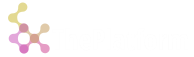🔍

## Table modification

Table amend/dmend work mostly the same as for dictionaries. Following example increases the "b" field by 1 for records at positions 0 and 2.

``````o)a:(+:)`a`b`c!(1 2 3;1 2 3;1 2 3); .[a;(`b;0 2);+;1]
a b c
-----
1 2 1
2 2 2
3 4 3
o)
``````

To create a new integer field of ones, do:

``````o)a:(+:)`a`b`c!(1 2 3;1 2 3;1 2 3); @[a;`d;:;1]
a b c d
-------
1 1 1 1
2 2 2 1
3 3 3 1
o)
``````

You can also combine primitive function application with creating a new field:

``````o)a:(+:)`a`b`c!(1 2 3;1 2 3;1 2 3); @[a;`a`b`d;+;1]
a b c d
-------
2 2 1 1
3 3 2 1
4 4 3 1
o)
``````

Multiplication also works:

``````o)a:(+:)(,`a)!,(1 2 3); @[a;`a`b;*;2]
a b
---
2 2
4 2
6 2
o)
``````

You can see that the "initial" value for addition and multiplication is different. Indeed, assign/plus/minus dyads assume zero value as initial, mul/div take one for initial.

... however, the following code won't work, as interpreter does not try to analyze lambda result types.

``````o)a:(+:)`a`b`c!(1 2 3;1 2 3;1 2 3); @[a;`a`b`d;{x+y};1]
** runtime error: `amend`:
fields: non-enumerable iterator
``````

More complex example shows creating of a new field and vector addition as single amend expression:

``````o)a:(+:)`a`b`c!(1 2 3;1 2 3;1 2 3); @[a;`a`b`d;+;(4 4 4;1;2)]
a b c d
-------
5 2 1 2
6 3 2 2
7 4 3 2
o)
``````

<<< prev Arithmetics
next >>> Inserts